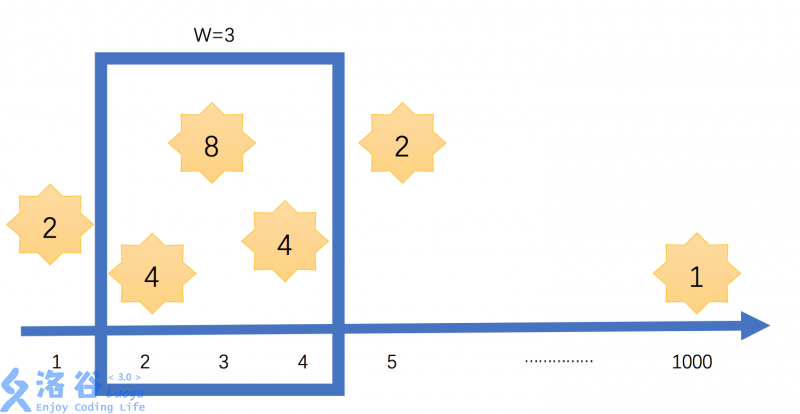6 3
1 2
2 4
3 8
4 4
5 2
1000 1

16

### 说明/提示### 题解代码

``````看到这个题目

"在你窗外闪耀的星星"

*/
include
using namespace std;
long long int a,b;
long long int ans;
void qsort(int x,int y)
{
int i=x,j=y;
int mid=b[(x+y)/2];
do
{
while(b[i]mid)
j--;
if(i<=j)         {             swap(a[i],a[j]);             swap(b[i],b[j]);             i++;j--;         }     }while(i<=j);     if(x>n>>m;
for(int i=1;i<=n;i++)     cin>>b[i]>>a[i];
qsort(1,n);
int j=1;
long long s=0;
for(int i=1;i<=n;i++)
{
s-=a[i-1];
while(j<=n and b[j]-b[i]<m)
{
s=a[j]+s;
j++;
}
ans=max(ans,s);
}
cout<<ans<<endl;
return 0;
}
//纵然千秋已逝
//愿你
//芳华初始``````